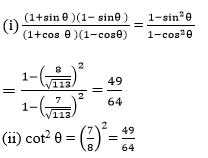Newbie

# If cot θ = 7/8, evaluate :(ii) cot2 θ .Q7(2)

• 0

Find this important question of  introduction to trigonometry of class 10 ncert . Sir  please help me to solve the exercise 8.1 question number 7(2), its very hard to solve   .   If cot θ = 7/8, evaluate :(ii) cot2 θ .

Share

1. Let us assume a △ABC in which ∠B = 90° and ∠C = θ

Given:

cot θ = BC/AB = 7/8

Let BC = 7k and AB = 8k, where k is a positive real number

According to Pythagoras theorem in △ABC we get.

AC2 = AB2+BC2

AC2 = (8k)2+(7k)2

AC2 = 64k2+49k2

AC2 = 113k2

AC = √113 k

According to the sine and cos function ratios, it is written as

sin θ = AB/AC = Opposite Side/Hypotenuse = 8k/√113 k = 8/√113 and

cos θ = Adjacent Side/Hypotenuse = BC/AC = 7k/√113 k = 7/√113

Now apply the values of sin function and cos function:• 0